# Extending ggplot2

This vignette documents the official extension mechanism provided in ggplot2 2.0.0. This vignette is a high-level adjunct to the low-level details found in `?Stat`, `?Geom` and `?theme`. You’ll learn how to extend ggplot2 by creating a new stat, geom, or theme.

As you read this document, you’ll see many things that will make you scratch your head and wonder why on earth is it designed this way? Mostly it’s historical accident - I wasn’t a terribly good R programmer when I started writing ggplot2 and I made a lot of questionable decisions. We cleaned up as many of those issues as possible in the 2.0.0 release, but some fixes simply weren’t worth the effort.

## ggproto

All ggplot2 objects are built using the ggproto system of object oriented programming. This OO system is used only in one place: ggplot2. This is mostly historical accident: ggplot2 started off using proto because I needed mutable objects. This was well before the creation of (the briefly lived) mutatr, reference classes and R6: proto was the only game in town.

But why ggproto? Well when we turned to add an official extension mechanism to ggplot2, we found a major problem that caused problems when proto objects were extended in a different package (methods were evaluated in ggplot2, not the package where the extension was added). We tried converting to R6, but it was a poor fit for the needs of ggplot2. We could’ve modified proto, but that would’ve first involved understanding exactly how proto worked, and secondly making sure that the changes didn’t affect other users of proto.

It’s strange to say, but this is a case where inventing a new OO system was actually the right answer to the problem! Fortunately Winston is now very good at creating OO systems, so it only took him a day to come up with ggproto: it maintains all the features of proto that ggplot2 needs, while allowing cross package inheritance to work.

Here’s a quick demo of ggproto in action:

``````A <- ggproto("A", NULL,
x = 1,
inc = function(self) {
self\$x <- self\$x + 1
}
)
A\$x
#>  1
A\$inc()
A\$x
#>  2
A\$inc()
A\$inc()
A\$x
#>  4``````

The majority of ggplot2 classes are immutable and static: the methods neither use nor modify state in the class. They’re mostly used as a convenient way of bundling related methods together.

To create a new geom or stat, you will just create a new ggproto that inherits from `Stat`, `Geom` and override the methods described below.

## Creating a new stat

### The simplest stat

We’ll start by creating a very simple stat: one that gives the convex hull (the c hull) of a set of points. First we create a new ggproto object that inherits from `Stat`:

``````StatChull <- ggproto("StatChull", Stat,
compute_group = function(data, scales) {
data[chull(data\$x, data\$y), , drop = FALSE]
},

required_aes = c("x", "y")
)``````

The two most important components are the `compute_group()` method (which does the computation), and the `required_aes` field, which lists which aesthetics must be present in order for the stat to work.

Next we write a layer function. Unfortunately, due to an early design mistake I called these either `stat_()` or `geom_()`. A better decision would have been to call them `layer_()` functions: that’s a more accurate description because every layer involves a stat and a geom.

All layer functions follow the same form - you specify defaults in the function arguments and then call the `layer()` function, sending `...` into the `params` argument. The arguments in `...` will either be arguments for the geom (if you’re making a stat wrapper), arguments for the stat (if you’re making a geom wrapper), or aesthetics to be set. `layer()` takes care of teasing the different parameters apart and making sure they’re stored in the right place:

``````stat_chull <- function(mapping = NULL, data = NULL, geom = "polygon",
position = "identity", na.rm = FALSE, show.legend = NA,
inherit.aes = TRUE, ...) {
layer(
stat = StatChull, data = data, mapping = mapping, geom = geom,
position = position, show.legend = show.legend, inherit.aes = inherit.aes,
params = list(na.rm = na.rm, ...)
)
}``````

(Note that if you’re writing this in your own package, you’ll either need to call `ggplot2::layer()` explicitly, or import the `layer()` function into your package namespace.)

Once we have a layer function we can try our new stat:

``````ggplot(mpg, aes(displ, hwy)) +
geom_point() +
stat_chull(fill = NA, colour = "black")``````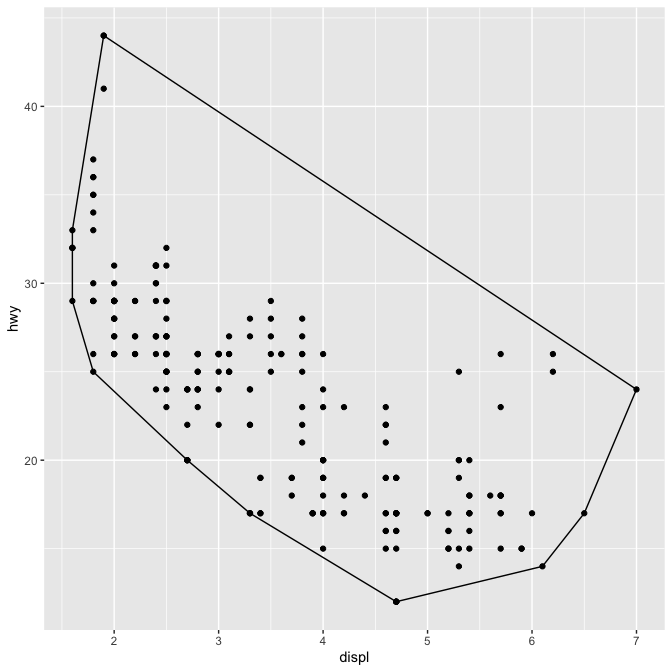(We’ll see later how to change the defaults of the geom so that you don’t need to specify `fill = NA` every time.)

Once we’ve written this basic object, ggplot2 gives a lot for free. For example, ggplot2 automatically preserves aesthetics that are constant within each group:

``````ggplot(mpg, aes(displ, hwy, colour = drv)) +
geom_point() +
stat_chull(fill = NA)``````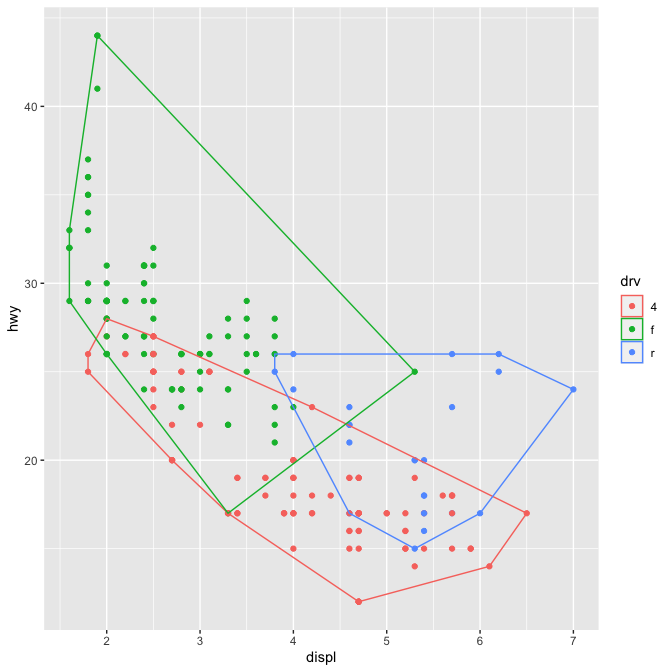We can also override the default geom to display the convex hull in a different way:

``````ggplot(mpg, aes(displ, hwy)) +
stat_chull(geom = "point", size = 4, colour = "red") +
geom_point()``````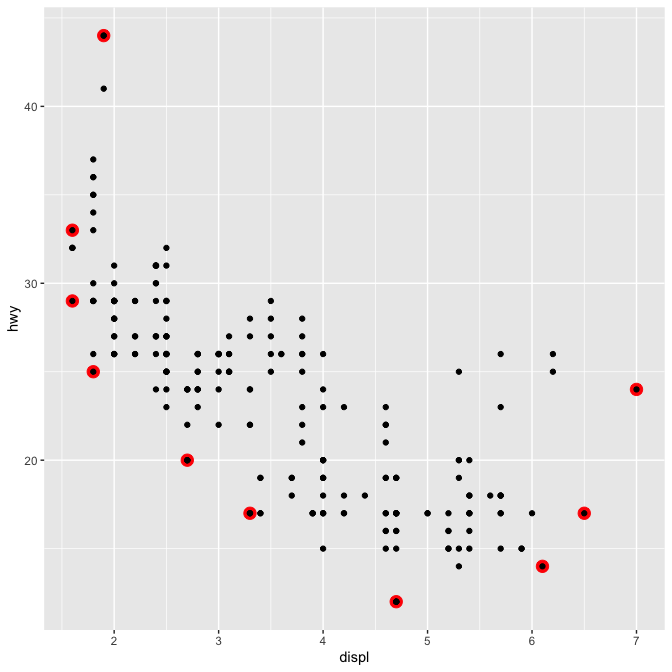### Stat parameters

A more complex stat will do some computation. Let’s implement a simple version of `geom_smooth()` that adds a line of best fit to a plot. We create a `StatLm` that inherits from `Stat` and a layer function, `stat_lm()`:

``````StatLm <- ggproto("StatLm", Stat,
required_aes = c("x", "y"),

compute_group = function(data, scales) {
rng <- range(data\$x, na.rm = TRUE)
grid <- data.frame(x = rng)

mod <- lm(y ~ x, data = data)
grid\$y <- predict(mod, newdata = grid)

grid
}
)

stat_lm <- function(mapping = NULL, data = NULL, geom = "line",
position = "identity", na.rm = FALSE, show.legend = NA,
inherit.aes = TRUE, ...) {
layer(
stat = StatLm, data = data, mapping = mapping, geom = geom,
position = position, show.legend = show.legend, inherit.aes = inherit.aes,
params = list(na.rm = na.rm, ...)
)
}

ggplot(mpg, aes(displ, hwy)) +
geom_point() +
stat_lm()``````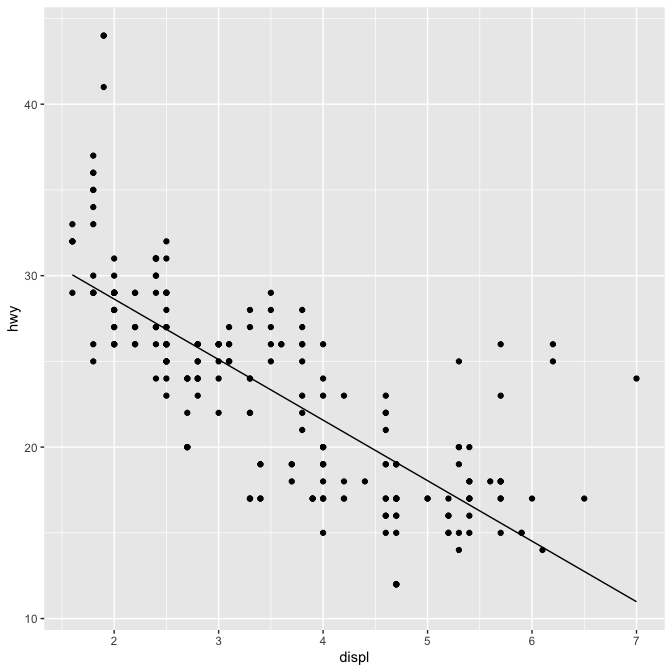`StatLm` is inflexible because it has no parameters. We might want to allow the user to control the model formula and the number of points used to generate the grid. To do so, we add arguments to the `compute_group()` method and our wrapper function:

``````StatLm <- ggproto("StatLm", Stat,
required_aes = c("x", "y"),

compute_group = function(data, scales, params, n = 100, formula = y ~ x) {
rng <- range(data\$x, na.rm = TRUE)
grid <- data.frame(x = seq(rng, rng, length = n))

mod <- lm(formula, data = data)
grid\$y <- predict(mod, newdata = grid)

grid
}
)

stat_lm <- function(mapping = NULL, data = NULL, geom = "line",
position = "identity", na.rm = FALSE, show.legend = NA,
inherit.aes = TRUE, n = 50, formula = y ~ x,
...) {
layer(
stat = StatLm, data = data, mapping = mapping, geom = geom,
position = position, show.legend = show.legend, inherit.aes = inherit.aes,
params = list(n = n, formula = formula, na.rm = na.rm, ...)
)
}

ggplot(mpg, aes(displ, hwy)) +
geom_point() +
stat_lm(formula = y ~ poly(x, 10)) +
stat_lm(formula = y ~ poly(x, 10), geom = "point", colour = "red", n = 20)``````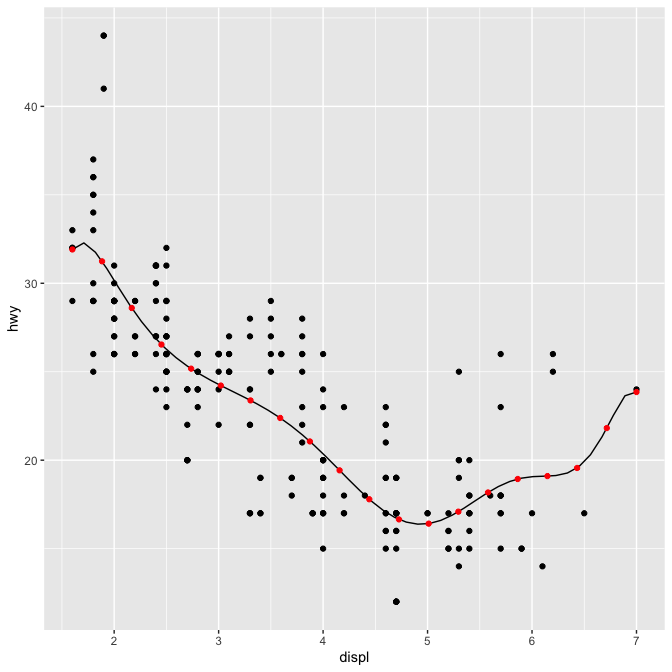Note that we don’t have to explicitly include the new parameters in the arguments for the layer, `...` will get passed to the right place anyway. But you’ll need to document them somewhere so the user knows about them. Here’s a brief example. Note `@inheritParams ggplot2::stat_identity`: that will automatically inherit documentation for all the parameters also defined for `stat_identity()`.

``````#' @export
#' @inheritParams ggplot2::stat_identity
#' @param formula The modelling formula passed to \code{lm}. Should only
#'   involve \code{y} and \code{x}
#' @param n Number of points used for interpolation.
stat_lm <- function(mapping = NULL, data = NULL, geom = "line",
position = "identity", na.rm = FALSE, show.legend = NA,
inherit.aes = TRUE, n = 50, formula = y ~ x,
...) {
layer(
stat = StatLm, data = data, mapping = mapping, geom = geom,
position = position, show.legend = show.legend, inherit.aes = inherit.aes,
params = list(n = n, formula = formula, na.rm = na.rm, ...)
)
}``````

`stat_lm()` must be exported if you want other people to use it. You could also consider exporting `StatLm` if you want people to extend the underlying object; this should be done with care.

### Picking defaults

Sometimes you have calculations that should be performed once for the complete dataset, not once for each group. This is useful for picking sensible default values. For example, if we want to do a density estimate, it’s reasonable to pick one bandwidth for the whole plot. The following Stat creates a variation of the `stat_density()` that picks one bandwidth for all groups by choosing the mean of the “best” bandwidth for each group (I have no theoretical justification for this, but it doesn’t seem unreasonable).

To do this we override the `setup_params()` method. It’s passed the data and a list of params, and returns an updated list.

``````StatDensityCommon <- ggproto("StatDensityCommon", Stat,
required_aes = "x",

setup_params = function(data, params) {
if (!is.null(params\$bandwidth))
return(params)

xs <- split(data\$x, data\$group)
bws <- vapply(xs, bw.nrd0, numeric(1))
bw <- mean(bws)
message("Picking bandwidth of ", signif(bw, 3))

params\$bandwidth <- bw
params
},

compute_group = function(data, scales, bandwidth = 1) {
d <- density(data\$x, bw = bandwidth)
data.frame(x = d\$x, y = d\$y)
}
)

stat_density_common <- function(mapping = NULL, data = NULL, geom = "line",
position = "identity", na.rm = FALSE, show.legend = NA,
inherit.aes = TRUE, bandwidth = NULL,
...) {
layer(
stat = StatDensityCommon, data = data, mapping = mapping, geom = geom,
position = position, show.legend = show.legend, inherit.aes = inherit.aes,
params = list(bandwidth = bandwidth, na.rm = na.rm, ...)
)
}

ggplot(mpg, aes(displ, colour = drv)) +
stat_density_common()
#> Picking bandwidth of 0.345``````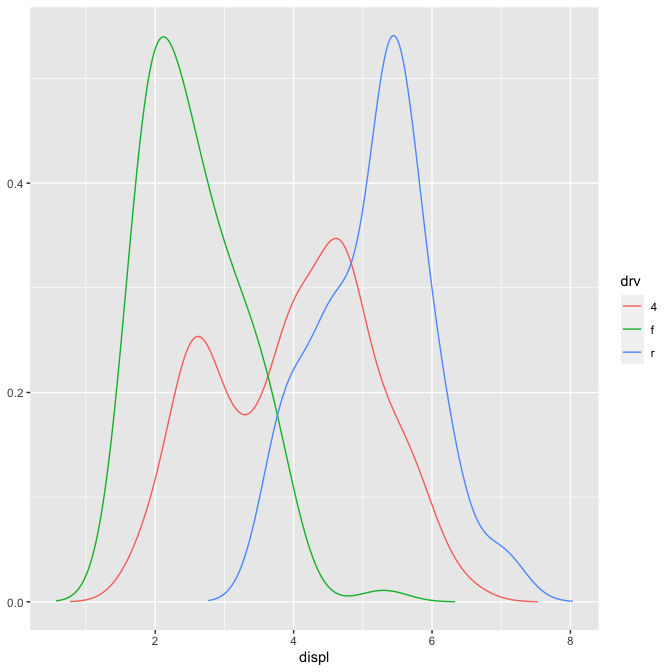``````
ggplot(mpg, aes(displ, colour = drv)) +
stat_density_common(bandwidth = 0.5)``````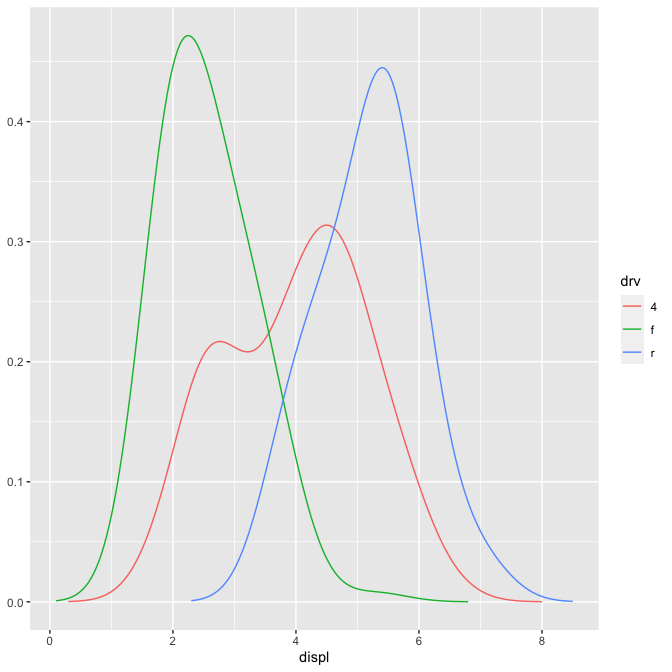I recommend using `NULL` as a default value. If you pick important parameters automatically, it’s a good idea to `message()` to the user (and when printing a floating point parameter, using `signif()` to show only a few significant digits).

### Variable names and default aesthetics

This stat illustrates another important point. If we want to make this stat usable with other geoms, we should return a variable called `density` instead of `y`. Then we can set up the `default_aes` to automatically map `density` to `y`, which allows the user to override it to use with different geoms:

``````StatDensityCommon <- ggproto("StatDensity2", Stat,
required_aes = "x",
default_aes = aes(y = stat(density)),

compute_group = function(data, scales, bandwidth = 1) {
d <- density(data\$x, bw = bandwidth)
data.frame(x = d\$x, density = d\$y)
}
)

ggplot(mpg, aes(displ, drv, colour = stat(density))) +
stat_density_common(bandwidth = 1, geom = "point")
#> Warning: `stat(density)` was deprecated in ggplot2 3.4.0.
#> This warning is displayed once every 8 hours.
#> Call `lifecycle::last_lifecycle_warnings()` to see where this warning was
#> generated.``````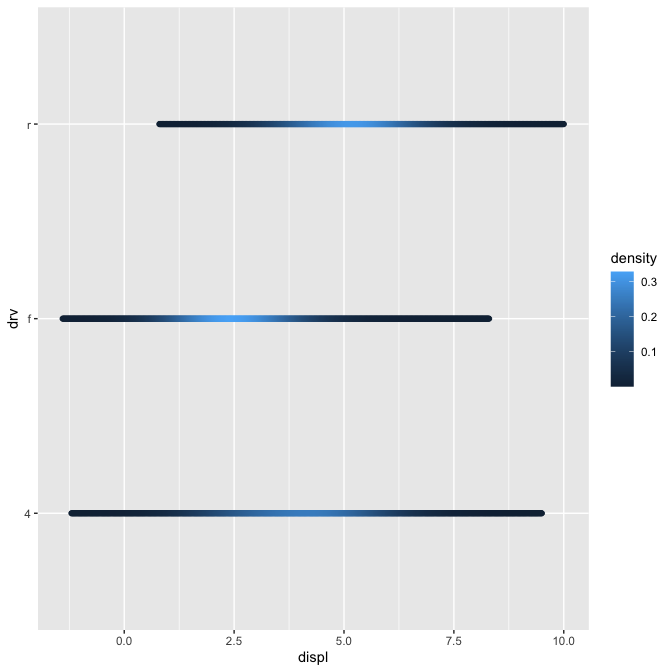However, using this stat with the area geom doesn’t work quite right. The areas don’t stack on top of each other:

``````ggplot(mpg, aes(displ, fill = drv)) +
stat_density_common(bandwidth = 1, geom = "area", position = "stack")``````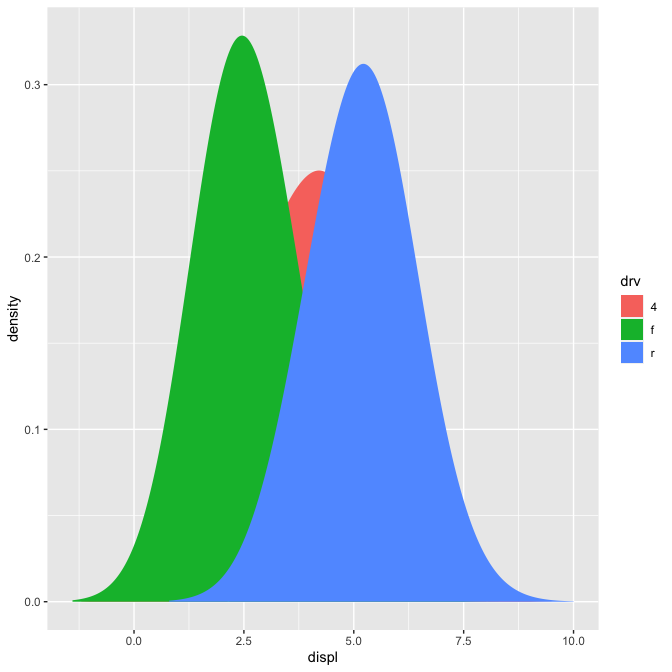This is because each density is computed independently, and the estimated `x`s don’t line up. We can resolve that issue by computing the range of the data once in `setup_params()`.

``````StatDensityCommon <- ggproto("StatDensityCommon", Stat,
required_aes = "x",
default_aes = aes(y = stat(density)),

setup_params = function(data, params) {
min <- min(data\$x) - 3 * params\$bandwidth
max <- max(data\$x) + 3 * params\$bandwidth

list(
bandwidth = params\$bandwidth,
min = min,
max = max,
na.rm = params\$na.rm
)
},

compute_group = function(data, scales, min, max, bandwidth = 1) {
d <- density(data\$x, bw = bandwidth, from = min, to = max)
data.frame(x = d\$x, density = d\$y)
}
)

ggplot(mpg, aes(displ, fill = drv)) +
stat_density_common(bandwidth = 1, geom = "area", position = "stack")``````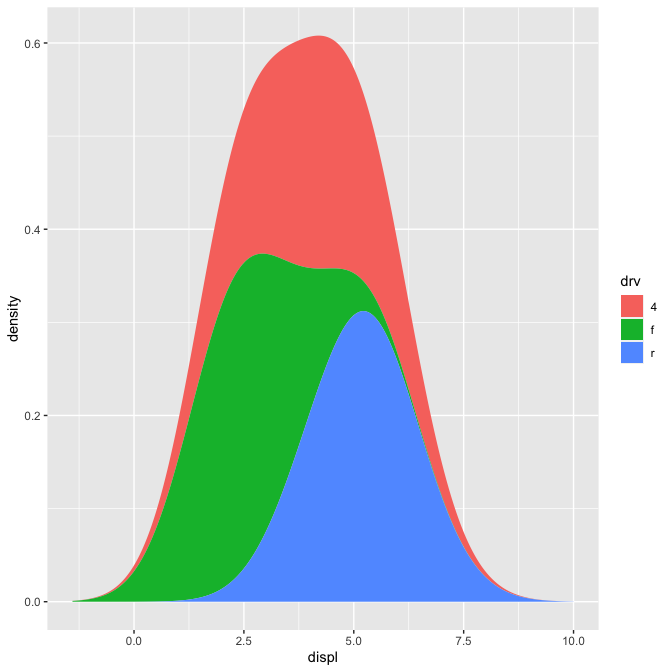``````ggplot(mpg, aes(displ, drv, fill = stat(density))) +
stat_density_common(bandwidth = 1, geom = "raster")``````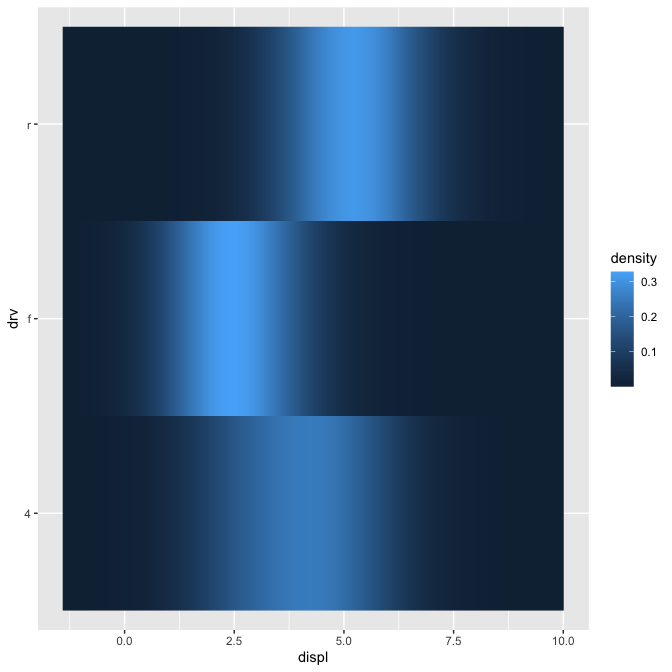### Exercises

1. Extend `stat_chull` to compute the alpha hull, as from the alphahull package. Your new stat should take an `alpha` argument.

2. Modify the final version of `StatDensityCommon` to allow the user to specify the `min` and `max` parameters. You’ll need to modify both the layer function and the `compute_group()` method.

Note: be careful when adding parameters to a layer function. The following names col, color, pch, cex, lty, lwd, srt, adj, bg, fg, min, and max are intentionally renamed to accomodate base graphical parameter names. For example, a value passed as min to a layer appears as ymin in the `setup_params` list of params. It is recommended you avoid using these names for layer parameters.

3. Compare and contrast `StatLm` to `ggplot2::StatSmooth`. What key differences make `StatSmooth` more complex than `StatLm`?

## Creating a new geom

It’s harder to create a new geom than a new stat because you also need to know some grid. ggplot2 is built on top of grid, so you’ll need to know the basics of drawing with grid. If you’re serious about adding a new geom, I’d recommend buying R graphics by Paul Murrell. It tells you everything you need to know about drawing with grid.

### A simple geom

It’s easiest to start with a simple example. The code below is a simplified version of `geom_point()`:

``````GeomSimplePoint <- ggproto("GeomSimplePoint", Geom,
required_aes = c("x", "y"),
default_aes = aes(shape = 19, colour = "black"),
draw_key = draw_key_point,

draw_panel = function(data, panel_params, coord) {
coords <- coord\$transform(data, panel_params)
grid::pointsGrob(
coords\$x, coords\$y,
pch = coords\$shape,
gp = grid::gpar(col = coords\$colour)
)
}
)

geom_simple_point <- function(mapping = NULL, data = NULL, stat = "identity",
position = "identity", na.rm = FALSE, show.legend = NA,
inherit.aes = TRUE, ...) {
layer(
geom = GeomSimplePoint, mapping = mapping,  data = data, stat = stat,
position = position, show.legend = show.legend, inherit.aes = inherit.aes,
params = list(na.rm = na.rm, ...)
)
}

ggplot(mpg, aes(displ, hwy)) +
geom_simple_point()``````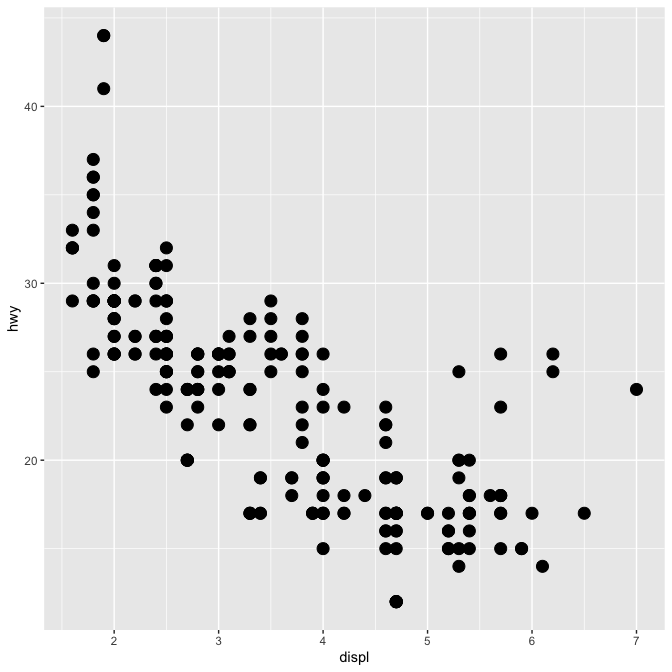This is very similar to defining a new stat. You always need to provide fields/methods for the four pieces shown above:

• `required_aes` is a character vector which lists all the aesthetics that the user must provide.

• `default_aes` lists the aesthetics that have default values.

• `draw_key` provides the function used to draw the key in the legend. You can see a list of all the build in key functions in `?draw_key`

• `draw_panel()` is where the magic happens. This function takes three arguments and returns a grid grob. It is called once for each panel. It’s the most complicated part and is described in more detail below.

`draw_panel()` has three arguments:

• `data`: a data frame with one column for each aesthetic.

• `panel_params`: a list of per-panel parameters generated by the coord. You should consider this an opaque data structure: don’t look inside it, just pass along to `coord` methods.

• `coord`: an object describing the coordinate system.

You need to use `panel_params` and `coord` together to transform the data `coords <- coord\$transform(data, panel_params)`. This creates a data frame where position variables are scaled to the range 0–1. You then take this data and call a grid grob function. (Transforming for non-Cartesian coordinate systems is quite complex - you’re best off transforming your data to the form accepted by an existing ggplot2 geom and passing it.)

### Collective geoms

Overriding `draw_panel()` is most appropriate if there is one graphic element per row. In other cases, you want graphic element per group. For example, take polygons: each row gives one vertex of a polygon. In this case, you should instead override `draw_group()`.

The following code makes a simplified version of `GeomPolygon`:

``````GeomSimplePolygon <- ggproto("GeomPolygon", Geom,
required_aes = c("x", "y"),

default_aes = aes(
colour = NA, fill = "grey20", linewidth = 0.5,
linetype = 1, alpha = 1
),

draw_key = draw_key_polygon,

draw_group = function(data, panel_params, coord) {
n <- nrow(data)
if (n <= 2) return(grid::nullGrob())

coords <- coord\$transform(data, panel_params)
# A polygon can only have a single colour, fill, etc, so take from first row
first_row <- coords[1, , drop = FALSE]

grid::polygonGrob(
coords\$x, coords\$y,
default.units = "native",
gp = grid::gpar(
col = first_row\$colour,
fill = scales::alpha(first_row\$fill, first_row\$alpha),
lwd = first_row\$linewidth * .pt,
lty = first_row\$linetype
)
)
}
)
geom_simple_polygon <- function(mapping = NULL, data = NULL, stat = "chull",
position = "identity", na.rm = FALSE, show.legend = NA,
inherit.aes = TRUE, ...) {
layer(
geom = GeomSimplePolygon, mapping = mapping, data = data, stat = stat,
position = position, show.legend = show.legend, inherit.aes = inherit.aes,
params = list(na.rm = na.rm, ...)
)
}

ggplot(mpg, aes(displ, hwy)) +
geom_point() +
geom_simple_polygon(aes(colour = class), fill = NA)``````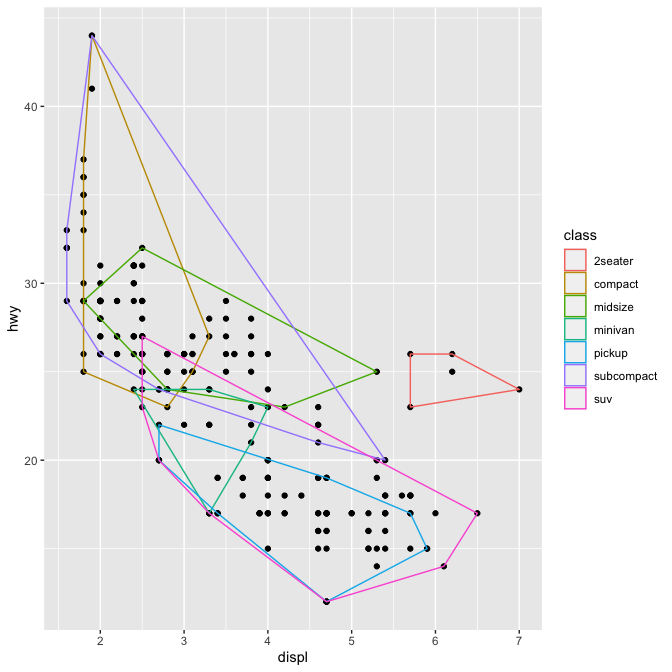There are a few things to note here:

• We override `draw_group()` instead of `draw_panel()` because we want one polygon per group, not one polygon per row.

• If the data contains two or fewer points, there’s no point trying to draw a polygon, so we return a `nullGrob()`. This is the graphical equivalent of `NULL`: it’s a grob that doesn’t draw anything and doesn’t take up any space.

• Note the units: `x` and `y` should always be drawn in “native” units. (The default units for `pointGrob()` is a native, so we didn’t need to change it there). `lwd` is measured in points, but ggplot2 uses mm, so we need to multiply it by the adjustment factor `.pt`.

You might want to compare this to the real `GeomPolygon`. You’ll see it overrides `draw_panel()` because it uses some tricks to make `polygonGrob()` produce multiple polygons in one call. This is considerably more complicated, but gives better performance.

### Inheriting from an existing Geom

Sometimes you just want to make a small modification to an existing geom. In this case, rather than inheriting from `Geom` you can inherit from an existing subclass. For example, we might want to change the defaults for `GeomPolygon` to work better with `StatChull`:

``````GeomPolygonHollow <- ggproto("GeomPolygonHollow", GeomPolygon,
default_aes = aes(colour = "black", fill = NA, linewidth = 0.5, linetype = 1,
alpha = NA)
)
geom_chull <- function(mapping = NULL, data = NULL,
position = "identity", na.rm = FALSE, show.legend = NA,
inherit.aes = TRUE, ...) {
layer(
stat = StatChull, geom = GeomPolygonHollow, data = data, mapping = mapping,
position = position, show.legend = show.legend, inherit.aes = inherit.aes,
params = list(na.rm = na.rm, ...)
)
}

ggplot(mpg, aes(displ, hwy)) +
geom_point() +
geom_chull()``````This doesn’t allow you to use different geoms with the stat, but that seems appropriate here since the convex hull is primarily a polygonal feature.

### Exercises

1. Compare and contrast `GeomPoint` with `GeomSimplePoint`.

2. Compare and contrast `GeomPolygon` with `GeomSimplePolygon`.

## Geoms and Stats with multiple orientation

Some layers have a specific orientation. `geom_bar()` e.g. have the bars along one axis, `geom_line()` will sort the input by one axis, etc. The original approach to using these geoms in the other orientation was to add `coord_flip()` to the plot to switch the position of the x and y axes. Following ggplot2 v3.3 all the geoms will natively work in both orientations without `coord_flip()`. The mechanism is that the layer will try to guess the orientation from the mapped data, or take direction from the user using the `orientation` parameter. To replicate this functionality in new stats and geoms there’s a few steps to take. We will look at the boxplot layer as an example instead of creating a new one from scratch.

### Omnidirectional stats

The actual guessing of orientation will happen in `setup_params()` using the `has_flipped_aes()` helper:

``````StatBoxplot\$setup_params
#> <ggproto method>
#>   <Wrapper function>
#>     function (...)
#> setup_params(..., self = self)
#>
#>   <Inner function (f)>
#>     function (self, data, params)
#> {
#>     params\$flipped_aes <- has_flipped_aes(data, params, main_is_orthogonal = TRUE,
#>         group_has_equal = TRUE, main_is_optional = TRUE)
#>     data <- flip_data(data, params\$flipped_aes)
#>     has_x <- !(is.null(data\$x) && is.null(params\$x))
#>     has_y <- !(is.null(data\$y) && is.null(params\$y))
#>     if (!has_x && !has_y) {
#>         cli::cli_abort("{.fn {snake_class(self)}} requires an {.field x} or {.field y} aesthetic.")
#>     }
#>     params\$width <- params\$width %||% (resolution(data\$x %||%
#>         0) * 0.75)
#>     if (!is_mapped_discrete(data\$x) && is.double(data\$x) && !has_groups(data) &&
#>         any(data\$x != data\$x[1L])) {
#>         cli::cli_warn(c("Continuous {.field {flipped_names(params\$flipped_aes)\$x}} aesthetic",
#>             i = "did you forget {.code aes(group = ...)}?"))
#>     }
#>     params
#> }``````

Following this is a call to `flip_data()` which will make sure the data is in horizontal orientation. The rest of the code can then simply assume that the data is in a specific orientation. The same thing happens in `setup_data()`:

``````StatBoxplot\$setup_data
#> <ggproto method>
#>   <Wrapper function>
#>     function (...)
#> setup_data(..., self = self)
#>
#>   <Inner function (f)>
#>     function (self, data, params)
#> {
#>     data <- flip_data(data, params\$flipped_aes)
#>     data\$x <- data\$x %||% 0
#>     data <- remove_missing(data, na.rm = params\$na.rm, vars = "x",
#>         name = "stat_boxplot")
#>     flip_data(data, params\$flipped_aes)
#> }``````

The data is flipped (if needed), manipulated, and flipped back as it is returned.

During the computation, this sandwiching between `flip_data()` is used as well, but right before the data is returned it will also get a `flipped_aes` column denoting if the data is flipped or not. This allows the stat to communicate to the geom that orientation has already been determined.

### Omnidirecitonal geoms

The setup for geoms is pretty much the same, with a few twists. `has_flipped_aes()` is also used in `setup_params()`, where it will usually be picked up from the `flipped_aes` column given by the stat. In `setup_data()` you will often see that `flipped_aes` is reassigned, to make sure it exist prior to position adjustment. This is needed if the geom is used together with a stat that doesn’t handle orientation (often `stat_identity()`):

``````GeomBoxplot\$setup_data
#> <ggproto method>
#>   <Wrapper function>
#>     function (...)
#> setup_data(...)
#>
#>   <Inner function (f)>
#>     function (data, params)
#> {
#>     data\$flipped_aes <- params\$flipped_aes
#>     data <- flip_data(data, params\$flipped_aes)
#>     data\$width <- data\$width %||% params\$width %||% (resolution(data\$x,
#>         FALSE) * 0.9)
#>     if (!is.null(data\$outliers)) {
#>         suppressWarnings({
#>             out_min <- vapply(data\$outliers, min, numeric(1))
#>             out_max <- vapply(data\$outliers, max, numeric(1))
#>         })
#>         data\$ymin_final <- pmin(out_min, data\$ymin)
#>         data\$ymax_final <- pmax(out_max, data\$ymax)
#>     }
#>     if (is.null(params) || is.null(params\$varwidth) || !params\$varwidth ||
#>         is.null(data\$relvarwidth)) {
#>         data\$xmin <- data\$x - data\$width/2
#>         data\$xmax <- data\$x + data\$width/2
#>     }
#>     else {
#>         data\$relvarwidth <- data\$relvarwidth/max(data\$relvarwidth)
#>         data\$xmin <- data\$x - data\$relvarwidth * data\$width/2
#>         data\$xmax <- data\$x + data\$relvarwidth * data\$width/2
#>     }
#>     data\$width <- NULL
#>     if (!is.null(data\$relvarwidth))
#>         data\$relvarwidth <- NULL
#>     flip_data(data, params\$flipped_aes)
#> }``````

In the `draw_*()` method you will once again sandwich any data manipulation between `flip_data()` calls. It is important to make sure that the data is flipped back prior to creating the grob or calling draw methods from other geoms.

### Dealing with required aesthetics

Omnidirectional layers usually have two different sets of required aesthetics. Which set is used is often how it knows the orientation. To handle this gracefully the `required_aes` field of `Stat` and `Geom` classes understands the `|` (or) operator. Looking at `GeomBoxplot` we can see how it is used:

``````GeomBoxplot\$required_aes
#>  "x|y"            "lower|xlower"   "upper|xupper"   "middle|xmiddle"
#>  "ymin|xmin"      "ymax|xmax"``````

This tells ggplot2 that either all the aesthetics before `|` are required or all the aesthetics after are required.

### Ambiguous layers

Some layers will not have a clear interpretation of their data in terms of orientation. A classic example is `geom_line()` which just by convention runs along the x-axis. There is nothing in the data itself that indicates that. For these geoms the user must indicate a flipped orientation by setting `orientation = "y"`. The stat or geom will then call `has_flipped_aes()` with `ambiguous = TRUE` to cancel any guessing based on data format. As an example we can see the `setup_params()` method of `GeomLine`:

``````GeomLine\$setup_params
#> <ggproto method>
#>   <Wrapper function>
#>     function (...)
#> setup_params(...)
#>
#>   <Inner function (f)>
#>     function (data, params)
#> {
#>     params\$flipped_aes <- has_flipped_aes(data, params, ambiguous = TRUE)
#>     params
#> }``````

If you’re going to create your own complete theme, there are a few things you need to know:

• Overriding existing elements, rather than modifying them
• The four global elements that affect (almost) every other theme element
• Complete vs. incomplete elements

### Overriding elements

By default, when you add a new theme element, it inherits values from the existing theme. For example, the following code sets the key colour to red, but it inherits the existing fill colour:

``````theme_grey()\$legend.key
#> List of 5
#>  \$ fill         : chr "grey95"
#>  \$ colour       : logi NA
#>  \$ linewidth    : NULL
#>  \$ linetype     : NULL
#>  \$ inherit.blank: logi TRUE
#>  - attr(*, "class")= chr [1:2] "element_rect" "element"

new_theme <- theme_grey() + theme(legend.key = element_rect(colour = "red"))
new_theme\$legend.key
#> List of 5
#>  \$ fill         : chr "grey95"
#>  \$ colour       : chr "red"
#>  \$ linewidth    : NULL
#>  \$ linetype     : NULL
#>  \$ inherit.blank: logi FALSE
#>  - attr(*, "class")= chr [1:2] "element_rect" "element"``````

To override it completely, use `%+replace%` instead of `+`:

``````new_theme <- theme_grey() %+replace% theme(legend.key = element_rect(colour = "red"))
new_theme\$legend.key
#> List of 5
#>  \$ fill         : NULL
#>  \$ colour       : chr "red"
#>  \$ linewidth    : NULL
#>  \$ linetype     : NULL
#>  \$ inherit.blank: logi FALSE
#>  - attr(*, "class")= chr [1:2] "element_rect" "element"``````

### Global elements

There are four elements that affect the global appearance of the plot:

Element Theme function Description
line `element_line()` all line elements
rect `element_rect()` all rectangular elements
text `element_text()` all text
title `element_text()` all text in title elements (plot, axes & legend)

These set default properties that are inherited by more specific settings. These are most useful for setting an overall “background” colour and overall font settings (e.g. family and size).

``````df <- data.frame(x = 1:3, y = 1:3)
base <- ggplot(df, aes(x, y)) +
geom_point() +
theme_minimal()

base``````